français | español | polski | 日本語 |

Pokemon Math 137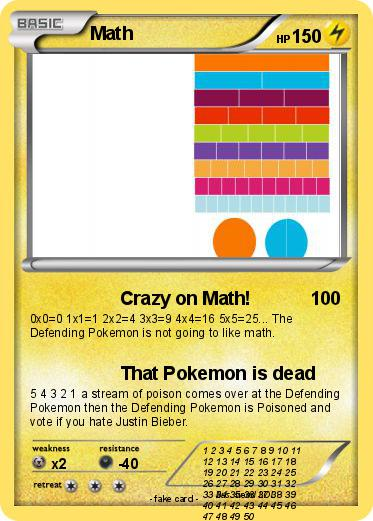Language Card : english

Creation Date : 29 February 2016

### Pokemon Passport

Name : Math

Serie : Black & White

Type : Lighting

Attack 1 : Crazy on Math!
0x0=0 1x1=1 2x2=4 3x3=9 4x4=16 5x5=25... The Defending Pokemon is not going to like math.

Attack 2 : That Pokemon is dead
5 4 3 2 1 a stream of poison comes over at the Defending Pokemon then the Defending Pokemon is Poisoned and vote if you hate Justin Bieber.

Comments : 1 2 3 4 5 6 7 8 9 10 11 12 13 14 15 16 17 18 19 20 21 22 23 24 25 26 27 28 29 30 31 32 33 34 35 36 37 38 39 40 41 42 43 44 45 46 47 48 49 50

Illustrator : weird LOL

### Vote for this card

(1 vote)

Related cards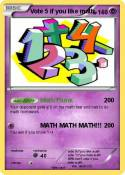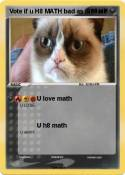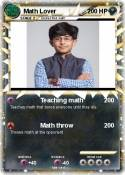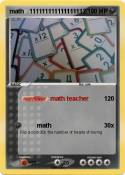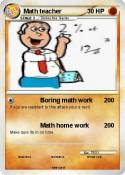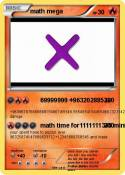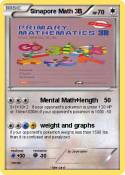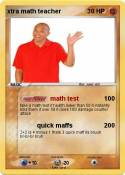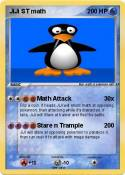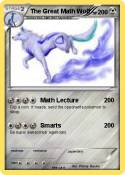Foxeh the math Fox | Khan academy | Vote 5 if you hate math | Math Mann | Ratey the Math Cat | I Can Do Math Everyday! | MATH | Math Teacher | MATh | math team | Math Book | Math knight | Brice | Math Teacher | Math :( | math 100 x | me in math | Math man | Daniel EX | Albert Einstein | MATH | MATH | Nerd | math | Math Homework | Cool Math | math doesn't lie | Tushar | Math 24 | Math Knight | Math Man | Math | Boring Math Teacher | Math | Mrs Ferriola | 1+1 | Ratey hte math cat | St Math | Mrs Baker | baldi | Math | Math | Math | prodigy | Math | Math | more math | math | Asian guy | Asian guy | School | Math | prodigy guy | extra math guy | xtramath guy | Math Quiz | Vote if you like math | Matt's math degree | ??????????????? | njo | Math | Pi | ??? | math | PIE | can you Math????? | math | Mr. C | Math | math pig